Published © GPL3+

# Arduino / ATtiny85 Delta AC 3-Phase Checker

This is a very simple way to control a 3-phase AC installation in a delta configuration.

BeginnerWork in progress4,866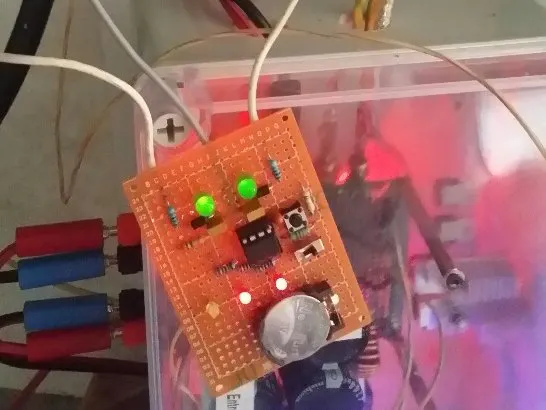## Things used in this project

### Hardware componentsArduino UNO
×15 mm LED: Red
×2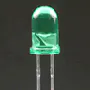5 mm LED: Green
×2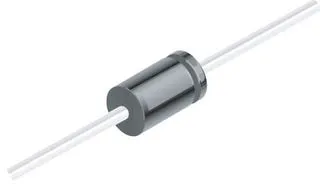1N4007 – High Voltage, High Current Rated Diode
×2
 resistors, diodes, capacitors.....
×1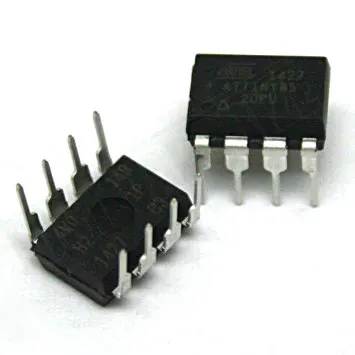Microchip Technology ATtiny85
×1

### Software apps and online servicesArduino IDE

### Hand tools and fabrication machinesSoldering iron (generic)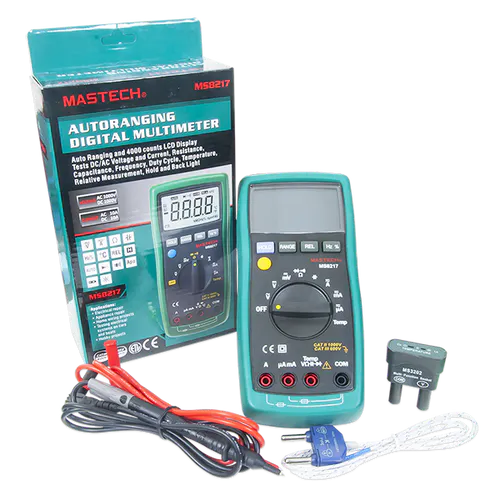Digilent Mastech MS8217 Autorange Digital Multimeter

Read more

## Custom parts and enclosures

### ATtiny85 3-phases checker ready for use

Here is an example of what can look like an ATtiny85 3-phases checker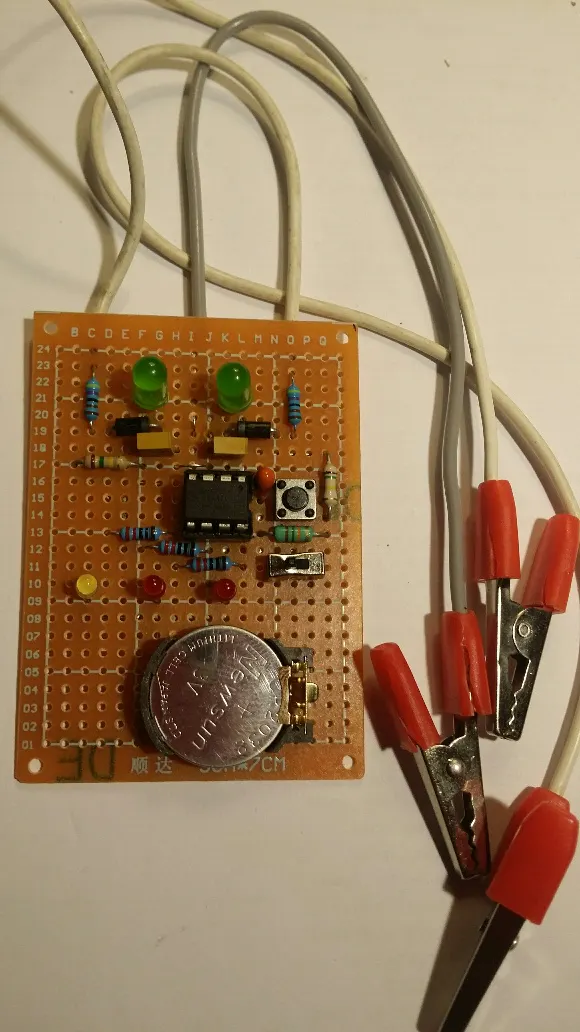## Schematics

### Arduino diagram for 3-phases checker

Arduino diagram for 3-phases checker.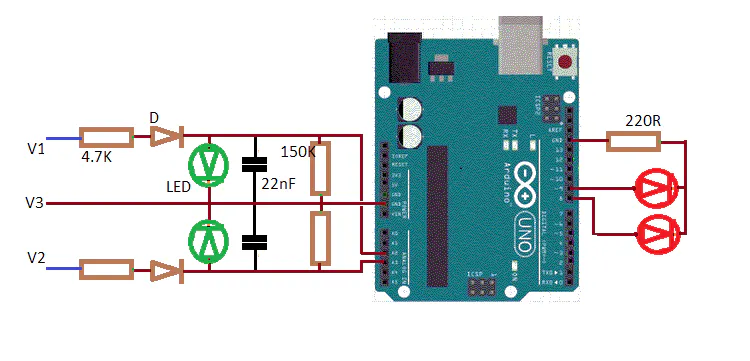### ATtiny85 3-phases checker diagram

ATtiny85 3-phases checker diagram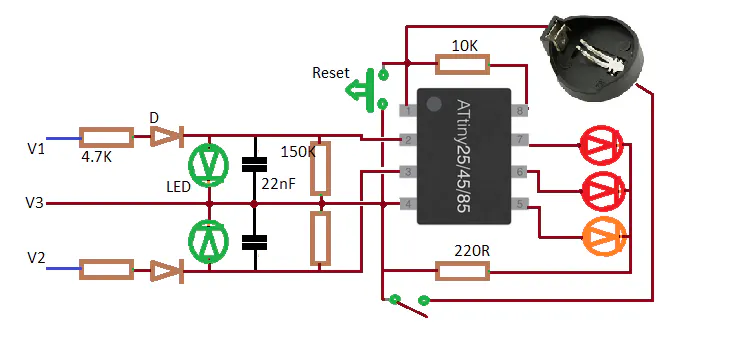## Code

### Arduino uno code for the 3-phases checker

C/C++
Arduino uno code for the 3-phases checker
```/* 3 phases checker in triangle configuration
*
*  For Arduino Uno
*
*

RESET  => Arduino Uno push-button
volt1 = A3  => 1st wire
volt2 = A2  => 2nd wire
volt3 = GND => 3rd wire
LED1 = 8   => 1st wire LED
LED2 = 9   => 2nd wire LED

*/

bool v1cut = false, v2cut = false;

void setup() {
pinMode(8, OUTPUT);
pinMode(9, OUTPUT);
}

void flasher(byte p) {
digitalWrite(p, HIGH);
delay(5);
digitalWrite(p, LOW);
}

void loop() {
unsigned long volt1, volt2;
byte nbre_cc;
byte a=0;

while( v1cut == false && v2cut == false ) {
volt1=0; volt2=0;
nbre_cc=0;
a++;
if( a%10 == 0 ) flasher(0);
for( byte i=0; i<100; i++ ) {
unsigned int v1 = analogRead(A3);
delayMicroseconds(10);
unsigned int v2 = analogRead(A2);
delayMicroseconds(10);
volt1 += v1; volt2 += v2;
if( v1-v2 < 20 || v2-v1 < 20 ) nbre_cc++;
delay(1);
}

if( nbre_cc > 50 ) { v1cut = true; v2cut = true; }
if( volt1 < 1000 ) v1cut = true;
if( volt2 < 1000 ) v2cut = true;
}   // end of while

if( v1cut == true ) flasher(2);
if( v2cut == true ) flasher(1);
}   // end of loop
```

### ATtiny85 3-phases checker code

C/C++
ATtiny85 3-phases checker code
```/* 3 phases checker in triangle configuration
*
*  For ATiny85
*
*
+-------+
RESET  <= PB5  1|*      |8  VCC
input1 <= A3 = PB3  2|       |7  PB2 => LED1
input2 <= A2 = PB4  3|       |6  PB1 => LED2
input3 <= GND  4|       |5  PB0 => startup LED
+-------+
connection :

RESET in PB5 = pin1  => push-button to GND // 10K to Vcc
volt1 in PB3 = pin2  => 1st wire
volt2 in PB4 = pin3  => 2nd wire
GND = pin4  => 3rd wire
LED1 in PB2 = pin7  => 1st wire
LED2 in PB1 = pin6  => 2nd wire
startup LED PB0 = pin5  => flash after each reset push

*/

#include <avr/sleep.h>
#include <EEPROM.h>

#ifndef cbi
#define cbi(sfr, bit) (_SFR_BYTE(sfr) &= ~_BV(bit))
#endif
#ifndef sbi
#define sbi(sfr, bit) (_SFR_BYTE(sfr) |= _BV(bit))
#endif

bool eveil = true;                      // WD flag
bool v1cut = false, v2cut = false;

void setup() {

pinMode(0, INPUT);
pinMode(3, INPUT);
pinMode(4, INPUT);
pinMode(2, INPUT);
pinMode(1, INPUT);

// the watchdog sets the interval delay :
// 0=16ms, 1=32ms,2=64ms, 3=128ms, 4=250ms, 5=500ms, 6=1s, 7=2s, 8=4s, 9=8s
setup_watchdog(6);
set_sleep_mode(SLEEP_MODE_PWR_DOWN);  // sleep mode

for( byte i=0; i<5; i++ ) {
flasher(0);
delay(100);
}
}

void flasher(byte p) {
pinMode(p, OUTPUT);
digitalWrite(p, HIGH);
delay(5);
digitalWrite(p, LOW);
pinMode(p, INPUT);
}

void loop() {

unsigned long volt1, volt2;
byte nbre_cc;
byte a=0;

while( v1cut == false && v2cut == false ) {
volt1=0; volt2=0;
nbre_cc=0;
a++;
if( a%10 == 0 ) flasher(0);
for( byte i=0; i<100; i++ ) {
unsigned int v1 = analogRead(A3);
delayMicroseconds(10);
unsigned int v2 = analogRead(A2);
delayMicroseconds(10);
volt1 += v1; volt2 += v2;
if( v1-v2 < 20 || v2-v1 < 20 ) nbre_cc++;
delay(1);
}

if( nbre_cc > 50 ) { v1cut = true; v2cut = true; }
if( volt1 < 1000 ) v1cut = true;
if( volt2 < 1000 ) v2cut = true;
}   // end of while

if( eveil == true ) {
eveil = false;
if( v1cut == true ) flasher(2);
if( v2cut == true ) flasher(1);
system_sleep();           // go to sleep for 8 secondes
}
}   // end of loop

// set system into the sleep state - system wakes up when wtchdog is timed out
void system_sleep() {
cbi(ADCSRA,ADEN);           // Switch ADC OFF, bacause ADC uses ~320uA
EEPROM.write(0, v1cut);
EEPROM.write(1, v2cut);
sleep_enable();
sleep_mode();               // System actually sleeps here
sleep_disable();            // System continues execution here when watchdog timed out
sbi(ADCSRA,ADEN);           // Switch Analog to Digital converter ON
v1cut = EEPROM.read(0);
v2cut = EEPROM.read(1);
}

void setup_watchdog( byte tempo ) {
byte val;
val = tempo & 7;
if (tempo > 7) val |= (1<<5);
val |= (1<<WDCE);
MCUSR &= ~(1<<WDRF);                  // start timed sequence
WDTCR |= (1<<WDCE) | (1<<WDE);        // set new watchdog timeout value
WDTCR = val;
WDTCR |= _BV(WDIE);
}

// Watchdog Interrupt Service / is executed when watchdog timed out
ISR(WDT_vect) { eveil = true; }        // set global flag
```